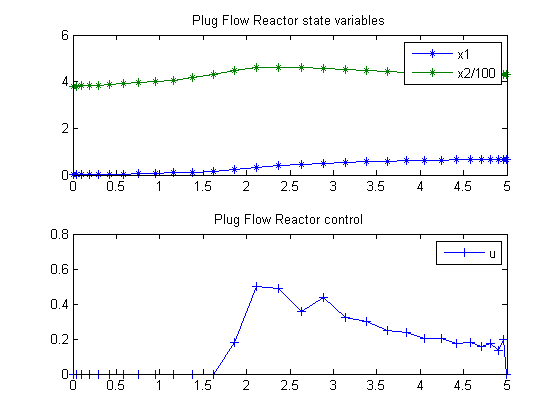87  Plug-Flow Tubular Reactor

A HYBRID METHOD FOR THE OPTIMAL CONTROL OF CHEMICAL PROCESSES 1998, E F Carrasco, J R Banga

Case Study II: Plug-Flow Tubular Reactor

87.1  Problem description

This case study considers a plug-flow reactor as studied by Reddy and Husain, Luus and Mekarapiruk and Luus. The objective is to maximize the normalized concentration of the desired product.

Find u(t) to maximize

 J = x1(tf)

subject to:

 dx1 dt
= (1−x1)*k1x1*k2
 dx2 dt
= 300*((1−x1)*k1x1*k2)−u*(x2−290)

where x1 denotes the normalized concentration of de desired product, and x2 is the temperature. The initial conditions are:

 x(t0) = [0  380]′

The rate constants are given by:

k1 = 1.7536e5*exp(−
 1.1374e4 1.9872*x2
k2 = 2.4885e10*exp(−
 2.2748e4 1.9872*x2

where the final time t_f = 5 min. The constraint on the control variable (the normalized coolant flow rate) is:

 0 <= u <= 0.5

In addition, there is an upper path constraint on the temperature:

 x2(t) <= 460

Reference: 

87.2  Problem setup

toms t
p = tomPhase('p', t, 0, 5, 30);
setPhase(p);

tomStates x1 x2
tomControls u

% Initial guess
x0 = {icollocate({x1 == 0.6*t/5
x2 == 380})
collocate(u == 0.25)};

% Box constraints
cbox = {0 <= icollocate(x1) <= 10
100 <= icollocate(x2) <= 460
0   <= collocate(u)   <= 0.5};

% Boundary constraints
cbnd = initial({x1 == 0; x2 == 380});

% ODEs and path constraints
k1 = 1.7536e5*exp(-1.1374e4/1.9872./x2);
k2 = 2.4885e10*exp(-2.2748e4/1.9872./x2);
ceq = collocate({
dot(x1) == (1-x1).*k1-x1.*k2
dot(x2) == 300*((1-x1).*k1-x1.*k2)-u.*(x2-290)});

% Objective
objective = -final(x1);

87.3  Solve the problem

options = struct;
options.name = 'Plug Flow Reactor';
solution = ezsolve(objective, {cbox, cbnd, ceq}, x0, options);
t  = subs(collocate(t),solution);
x1 = subs(collocate(x1),solution);
x2 = subs(collocate(x2),solution);
u  = subs(collocate(u),solution);
Problem type appears to be: lpcon
Starting numeric solver
===== * * * =================================================================== * * *
TOMLAB - Tomlab Optimization Inc. Development license  999001. Valid to 2011-02-05
=====================================================================================
Problem: ---  1: Plug Flow Reactor              f_k      -0.677125737913556680
sum(|constr|)      0.000031724693791993
f(x_k) + sum(|constr|)     -0.677094013219764700
f(x_0)     -0.599999999999999200

Solver: snopt.  EXIT=0.  INFORM=1.
SNOPT 7.2-5 NLP code
Optimality conditions satisfied

FuncEv    1 ConstrEv  346 ConJacEv  346 Iter  112 MinorIter  325
CPU time: 0.796875 sec. Elapsed time: 0.813000 sec.

87.4  Plot result

subplot(2,1,1)
plot(t,x1,'*-',t,x2/100,'*-');
legend('x1','x2/100');
title('Plug Flow Reactor state variables');

subplot(2,1,2)
plot(t,u,'+-');
legend('u');
title('Plug Flow Reactor control');